# MPI derived datatypes Edgar Gabriel Derived Datatypes Basic

• Slides: 38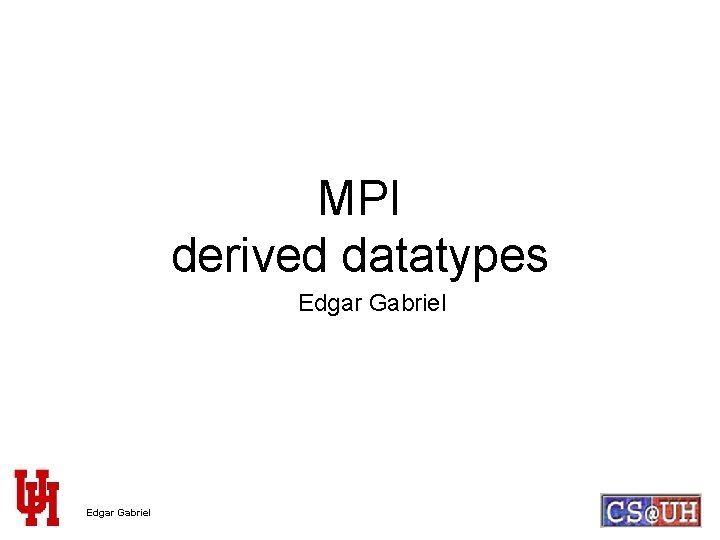MPI derived datatypes Edgar Gabriel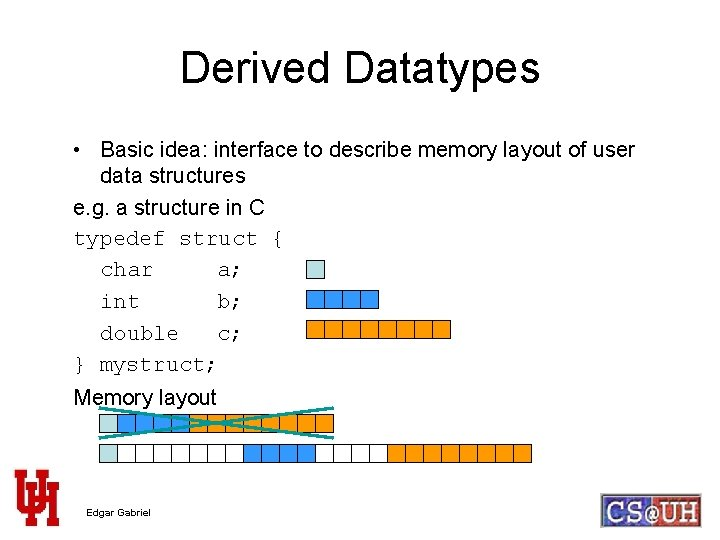Derived Datatypes • Basic idea: interface to describe memory layout of user data structures e. g. a structure in C typedef struct { char a; int b; double c; } mystruct; Memory layout Edgar Gabriel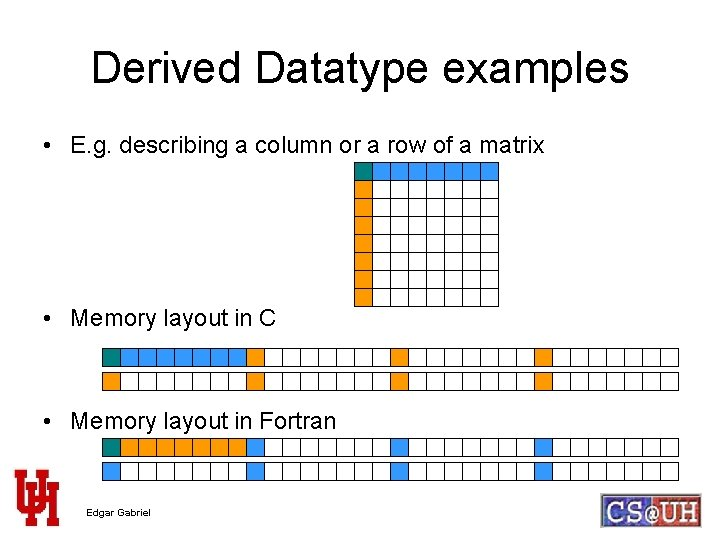Derived Datatype examples • E. g. describing a column or a row of a matrix • Memory layout in C • Memory layout in Fortran Edgar GabrielHow to describe noncontiguous data structures typedef struct { char a; int b; double c; } mystruct; baseaddr gap 1 address 2 gap 2 • using a list-I/O interface, e. g. <address, size> <baseaddr, sizeof(char)> <address 1, sizeof(int)> <address 2, sizeof(double)> • or <baseaddr, sizeof(char)> <baseaddr+gap 1, sizeof(int)> <baseaddr+gap 2, sizeof(double)> Edgar Gabriel…or in MPI terminology… • a list of <address, count, datatype> sequences <baseaddr, 1, MPI_CHAR> <baseaddr+gap 1, 1, MPI_INT> <baseaddr+gap 2, 1, MPI_DOUBLE> • …leading to the following interface… MPI_Type_struct (int count, int blocklength[], MPI_Aint displacements[], MPI_Datatype datatypes[], MPI_Datatype *newtype ); MPI_Type_create_struct (int count, int blocklength[], MPI_Aint displacements[], MPI_Datatype datatypes[], MPI_Datatype *newtype ); Edgar Gabriel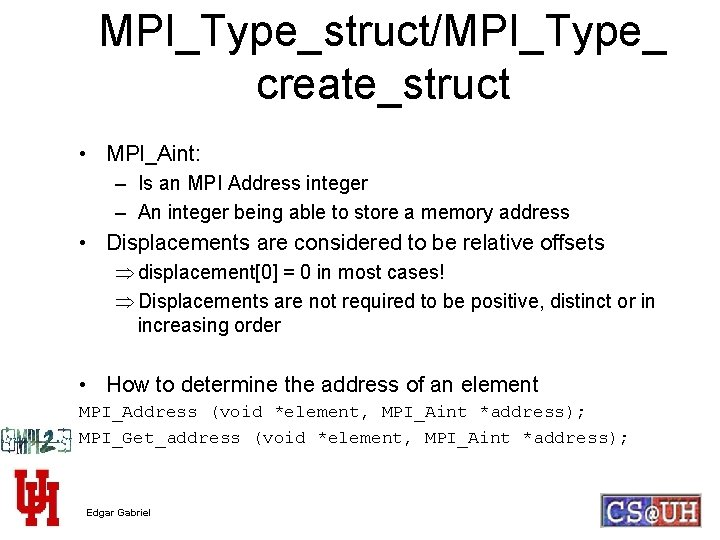MPI_Type_struct/MPI_Type_ create_struct • MPI_Aint: – Is an MPI Address integer – An integer being able to store a memory address • Displacements are considered to be relative offsets Þ displacement = 0 in most cases! Þ Displacements are not required to be positive, distinct or in increasing order • How to determine the address of an element MPI_Address (void *element, MPI_Aint *address); MPI_Get_address (void *element, MPI_Aint *address); Edgar GabrielAddresses in MPI • Why not use the & operator in C ? – ANSI C does NOT require that the value of the pointer returned by & is the absolute address of the object! – Might lead to problems in segmented memory space – Usually not a problem • In Fortran: all data elements passed to a single MPI_Type_struct call have to be in the same common block Edgar Gabriel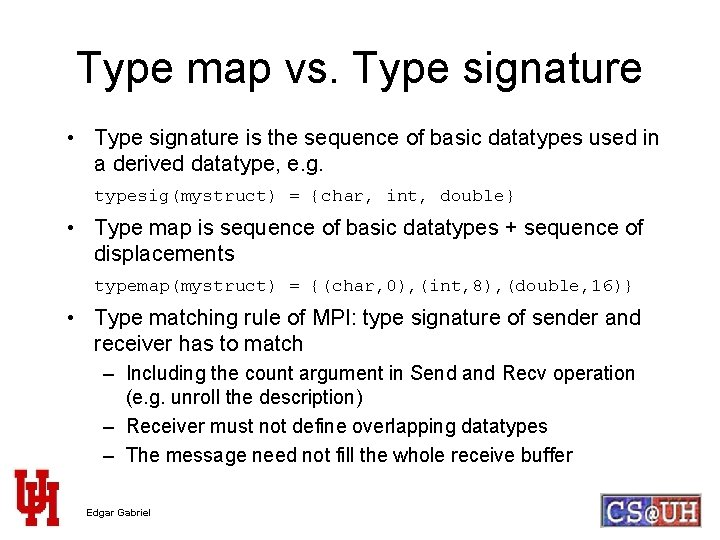Type map vs. Type signature • Type signature is the sequence of basic datatypes used in a derived datatype, e. g. typesig(mystruct) = {char, int, double} • Type map is sequence of basic datatypes + sequence of displacements typemap(mystruct) = {(char, 0), (int, 8), (double, 16)} • Type matching rule of MPI: type signature of sender and receiver has to match – Including the count argument in Send and Recv operation (e. g. unroll the description) – Receiver must not define overlapping datatypes – The message need not fill the whole receive buffer Edgar Gabriel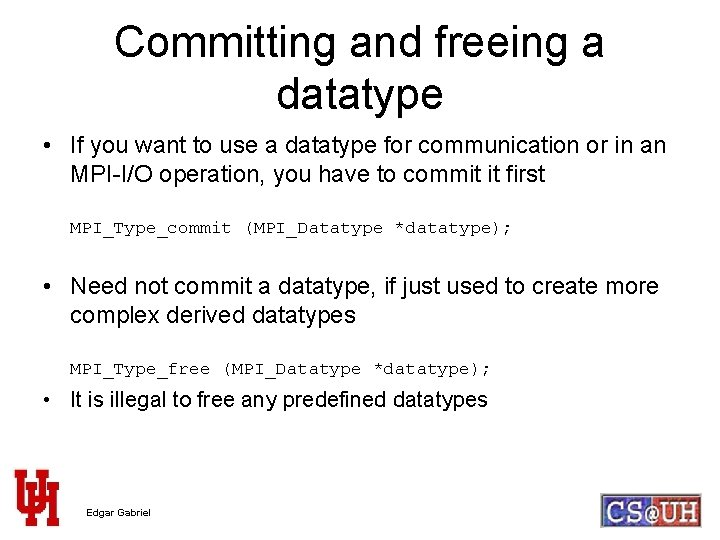Committing and freeing a datatype • If you want to use a datatype for communication or in an MPI-I/O operation, you have to commit it first MPI_Type_commit (MPI_Datatype *datatype); • Need not commit a datatype, if just used to create more complex derived datatypes MPI_Type_free (MPI_Datatype *datatype); • It is illegal to free any predefined datatypes Edgar Gabriel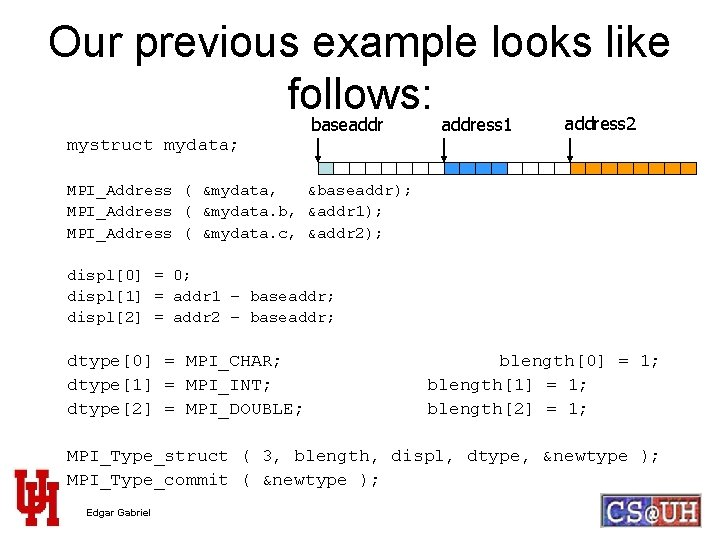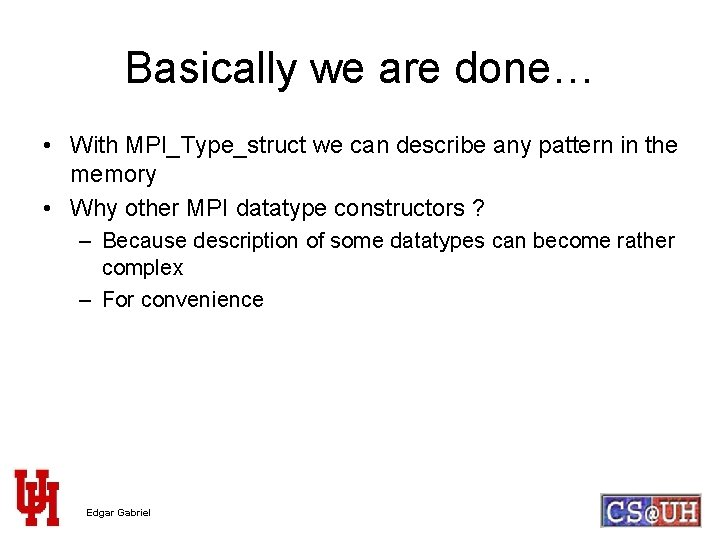Basically we are done… • With MPI_Type_struct we can describe any pattern in the memory • Why other MPI datatype constructors ? – Because description of some datatypes can become rather complex – For convenience Edgar GabrielMPI_Type_contiguous ( int count, MPI_Datatype datatype, MPI_Datatype *newtype ); • count elements of the same datatype forming a contiguous chunk in the memory int myvec; MPI_Type_contiguous ( 4, MPI_INT, &mybrandnewdatatype); MPI_Type_commit ( &mybrandnewdatatype ); MPI_Send ( myvec, 1, mybrandnewdatatype, … ); • Input datatype can be a derived datatype – End of one element of the derived datatype has to be exactly at the beginning of the next element of the derived datatype Edgar GabrielMPI_Type_vector( int count, int blocklength, int stride, MPI_Datatype datatype, MPI_Datatype *newtype ); • count blocks of blocklength elements of the same datatype • Between the start of each block there are stride elements of the same datatype stride=3 blocklength=2 Edgar Gabriel count=3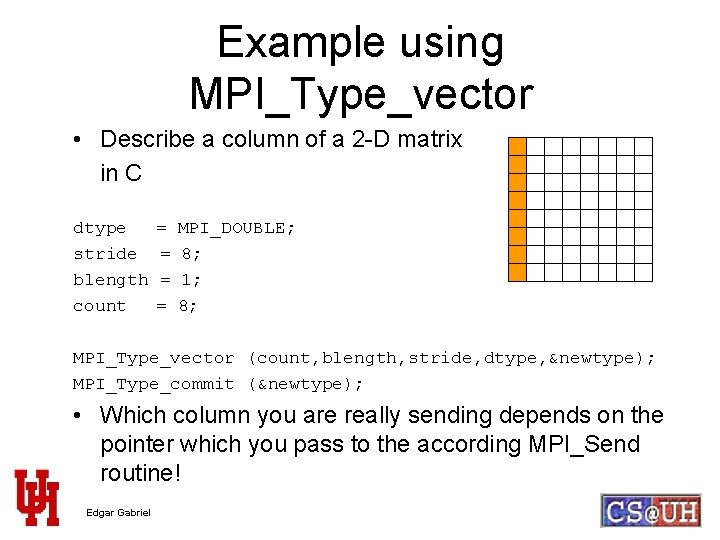Example using MPI_Type_vector • Describe a column of a 2 -D matrix in C dtype stride blength count = = MPI_DOUBLE; 8; 1; 8; MPI_Type_vector (count, blength, stride, dtype, &newtype); MPI_Type_commit (&newtype); • Which column you are really sending depends on the pointer which you pass to the according MPI_Send routine! Edgar Gabriel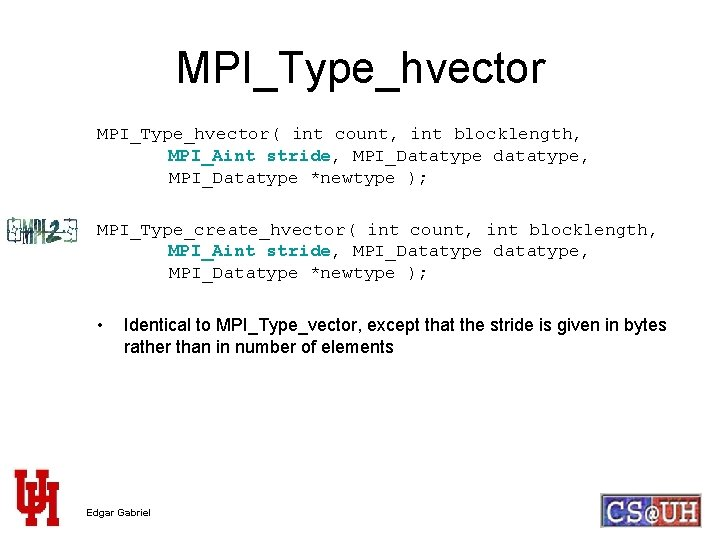MPI_Type_hvector( int count, int blocklength, MPI_Aint stride, MPI_Datatype datatype, MPI_Datatype *newtype ); MPI_Type_create_hvector( int count, int blocklength, MPI_Aint stride, MPI_Datatype datatype, MPI_Datatype *newtype ); • Identical to MPI_Type_vector, except that the stride is given in bytes rather than in number of elements Edgar Gabriel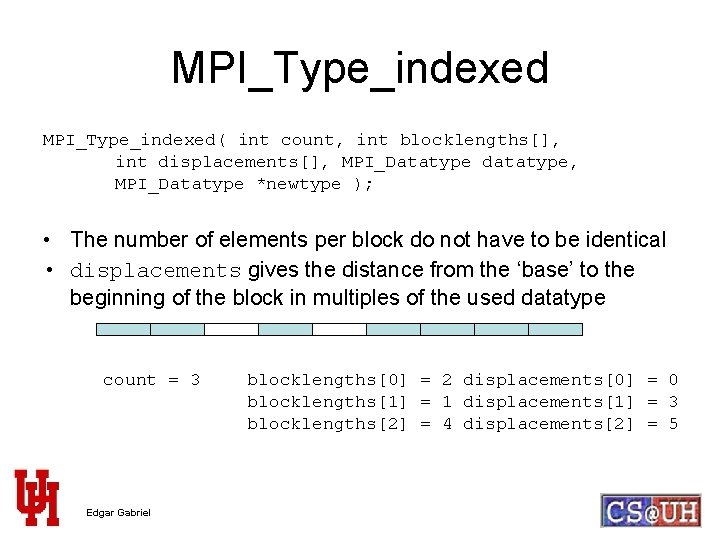MPI_Type_indexed( int count, int blocklengths[], int displacements[], MPI_Datatype datatype, MPI_Datatype *newtype ); • The number of elements per block do not have to be identical • displacements gives the distance from the ‘base’ to the beginning of the block in multiples of the used datatype count = 3 Edgar Gabriel blocklengths = 2 displacements = 0 blocklengths = 1 displacements = 3 blocklengths = 4 displacements = 5MPI_Type_hindexed( int count, int blocklengths[], MPI_Aint displacements[], MPI_Datatype datatype, MPI_Datatype *newtype ); MPI_Type_create_hindexed( int count, int blocklengths[], MPI_Aint displacements[], MPI_Datatype datatype, MPI_Datatype *newtype ); • Identical to MPI_Type_indexed, except that the displacements are given in bytes and not in multiples of the datatypes Edgar Gabriel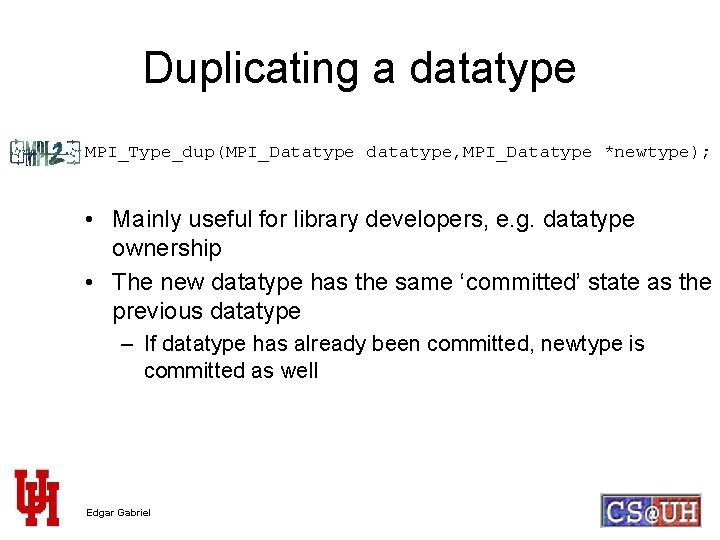Duplicating a datatype MPI_Type_dup(MPI_Datatype datatype, MPI_Datatype *newtype); • Mainly useful for library developers, e. g. datatype ownership • The new datatype has the same ‘committed’ state as the previous datatype – If datatype has already been committed, newtype is committed as well Edgar Gabriel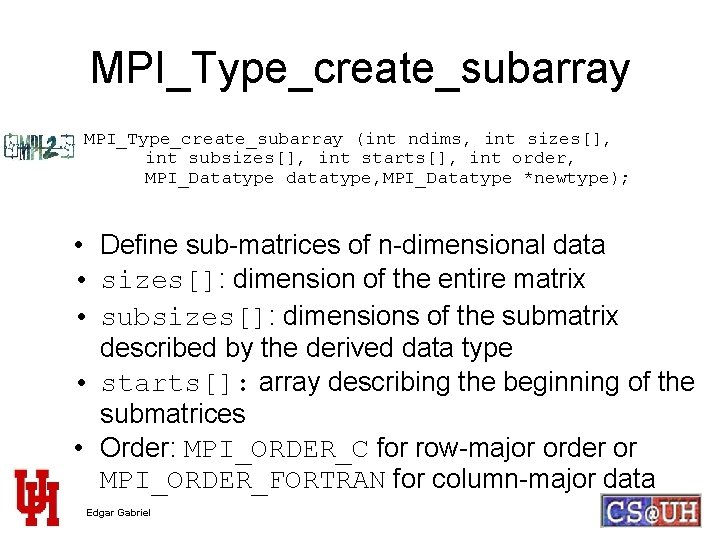MPI_Type_create_subarray (int ndims, int sizes[], int subsizes[], int starts[], int order, MPI_Datatype datatype, MPI_Datatype *newtype); • Define sub-matrices of n-dimensional data • sizes[]: dimension of the entire matrix • subsizes[]: dimensions of the submatrix described by the derived data type • starts[]: array describing the beginning of the submatrices • Order: MPI_ORDER_C for row-major order or MPI_ORDER_FORTRAN for column-major data Edgar Gabriel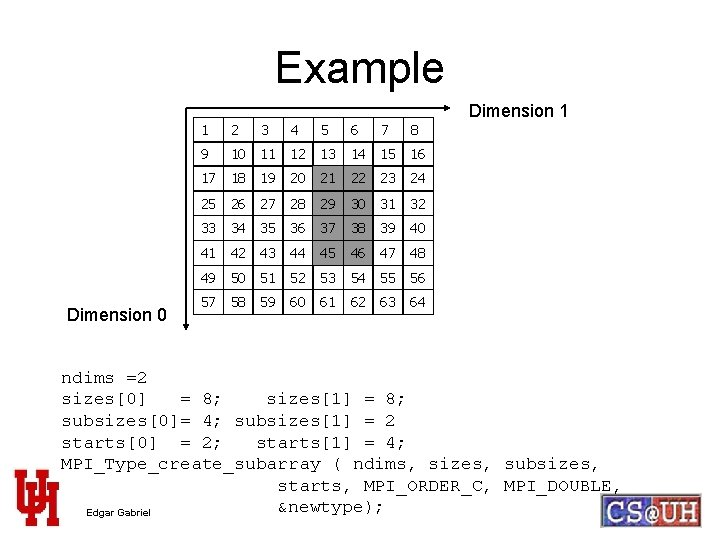Example Dimension 0 1 2 3 4 5 6 7 8 9 10 11 12 13 14 15 16 17 18 19 20 21 22 23 24 25 26 27 28 29 30 31 32 33 34 35 36 37 38 39 40 41 42 43 44 45 46 47 48 49 50 51 52 53 54 55 56 57 58 59 60 61 62 63 64 Dimension 1 ndims =2 sizes = 8; sizes = 8; subsizes= 4; subsizes = 2 starts = 2; starts = 4; MPI_Type_create_subarray ( ndims, sizes, subsizes, starts, MPI_ORDER_C, MPI_DOUBLE, &newtype); Edgar GabrielMore datatype constructors MPI_Type_create_darray(int size, int rank, int ndims, int gsizes[], int distribs[], int dargs[], int psizes[], int order, MPI_Datatype datatype, MPI_Datatype *newtype); • Describe HPF-like data distributions MPI_Type_create_indexed_block( int count, int blocklength, int displs[], MPI_Datatype datatype, MPI_Datatype *newtype ); • Further simplification of MPI_Type_indexed Edgar GabrielPortable vs. non-portable datatypes • Any data type constructors using byte-offsets are considered non-portable – Might rely on data alignment rules given on various platforms • Non-portable datatype constructors: – MPI_Type_struct – MPI_Type_hvector/MPI_Type_create_hvector – MPI_Type_hindexed/MPI_Type_create_hindexed • Non-portable datatypes are not allowed to be used in – one-sided operations – parallel File I/O operations Edgar GabrielA problem with the specification up to now typedef struct { char a; int b; double c; float d; } mystruct; mystruct mydata; • …but just want to send b and c of the structure, however multiple elements of mystruct Edgar Gabriel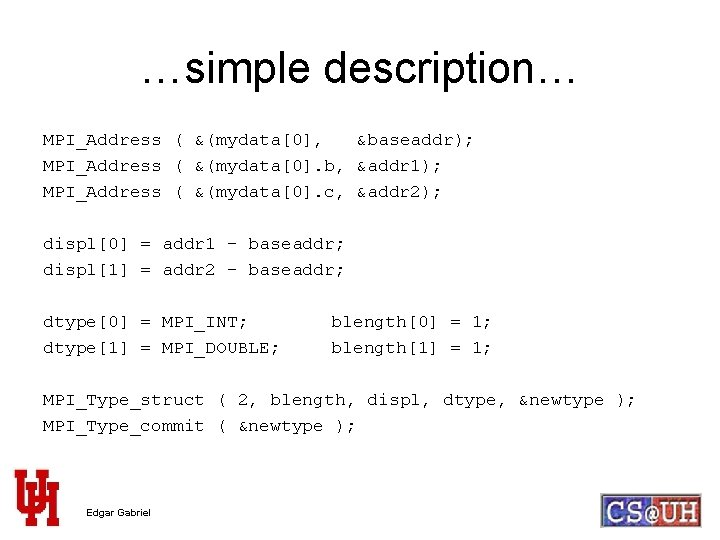…simple description… MPI_Address ( &(mydata, &baseaddr); MPI_Address ( &(mydata. b, &addr 1); MPI_Address ( &(mydata. c, &addr 2); displ = addr 1 - baseaddr; displ = addr 2 – baseaddr; dtype = MPI_INT; dtype = MPI_DOUBLE; blength = 1; blength = 1; MPI_Type_struct ( 2, blength, displ, dtype, &newtype ); MPI_Type_commit ( &newtype ); Edgar Gabriel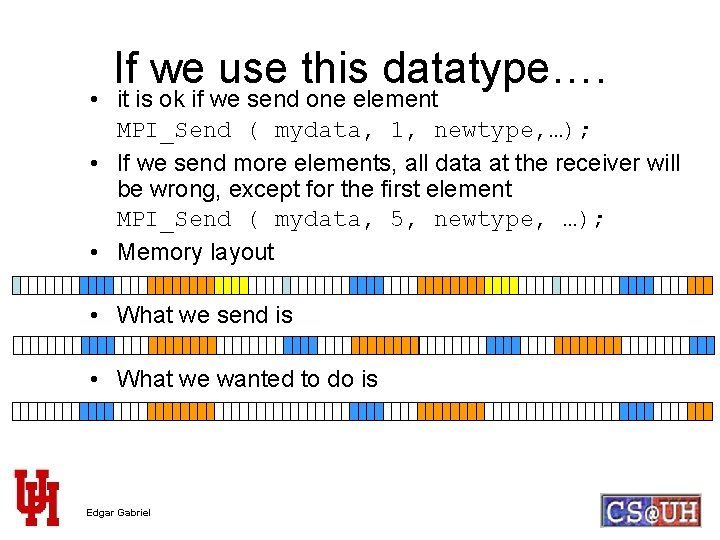If we use this datatype…. • it is ok if we send one element MPI_Send ( mydata, 1, newtype, …); • If we send more elements, all data at the receiver will be wrong, except for the first element MPI_Send ( mydata, 5, newtype, …); • Memory layout • What we send is • What we wanted to do is Edgar Gabriel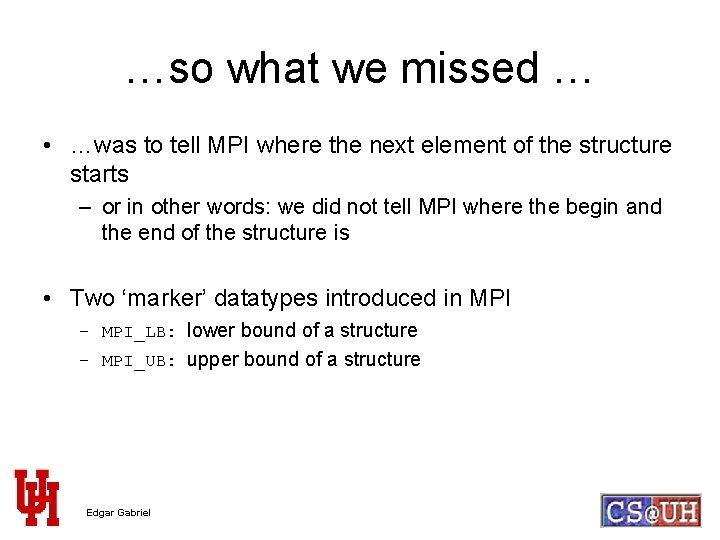…so what we missed … • …was to tell MPI where the next element of the structure starts – or in other words: we did not tell MPI where the begin and the end of the structure is • Two ‘marker’ datatypes introduced in MPI – MPI_LB: lower bound of a structure – MPI_UB: upper bound of a structure Edgar Gabriel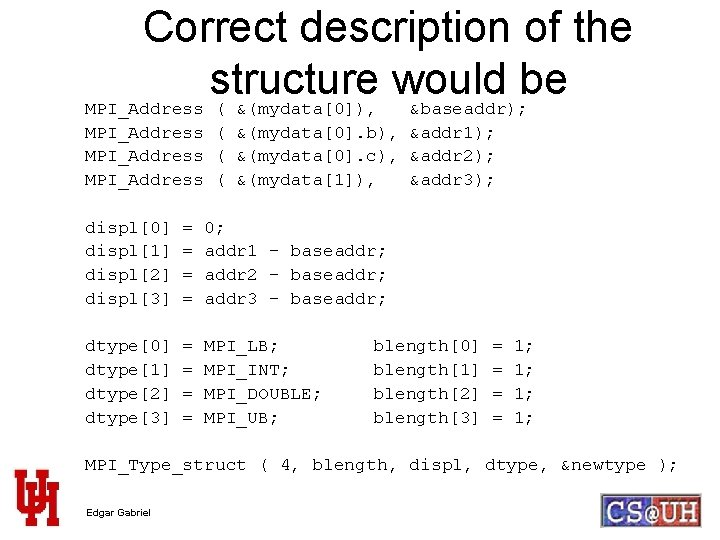Determining upper- and lower bound • Two functions to extract the upper and the lower bound of a datatype MPI_Type_ub ( MPI_Datatype dat, MPI_Aint *ub ); MPI_Type_lb ( MPI_Datatype dat, MPI_Aint *lb ); Edgar Gabriel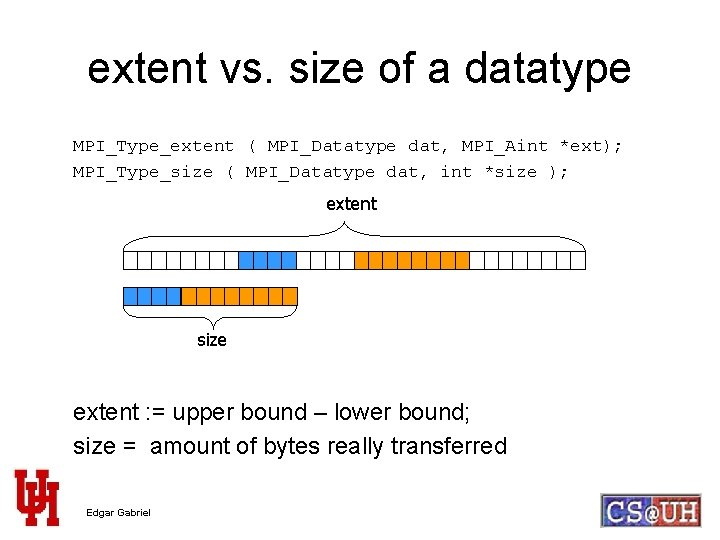extent vs. size of a datatype MPI_Type_extent ( MPI_Datatype dat, MPI_Aint *ext); MPI_Type_size ( MPI_Datatype dat, int *size ); extent size extent : = upper bound – lower bound; size = amount of bytes really transferred Edgar Gabriel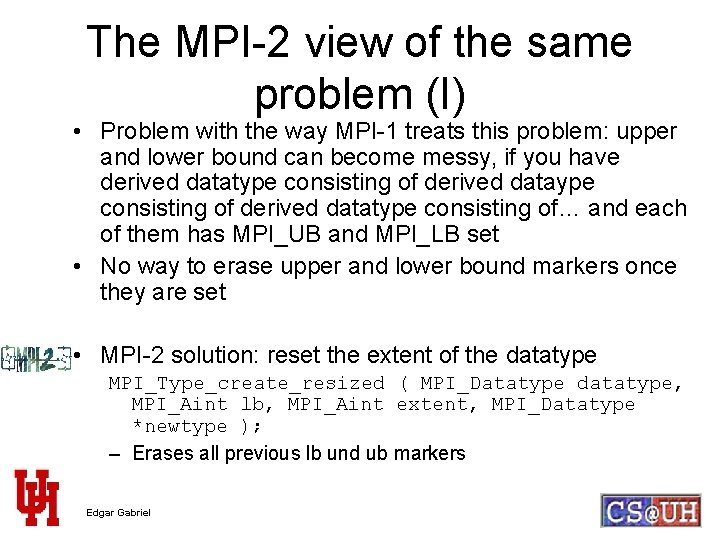The MPI-2 view of the same problem (I) • Problem with the way MPI-1 treats this problem: upper and lower bound can become messy, if you have derived datatype consisting of… and each of them has MPI_UB and MPI_LB set • No way to erase upper and lower bound markers once they are set • MPI-2 solution: reset the extent of the datatype MPI_Type_create_resized ( MPI_Datatype datatype, MPI_Aint lb, MPI_Aint extent, MPI_Datatype *newtype ); – Erases all previous lb und ub markers Edgar Gabriel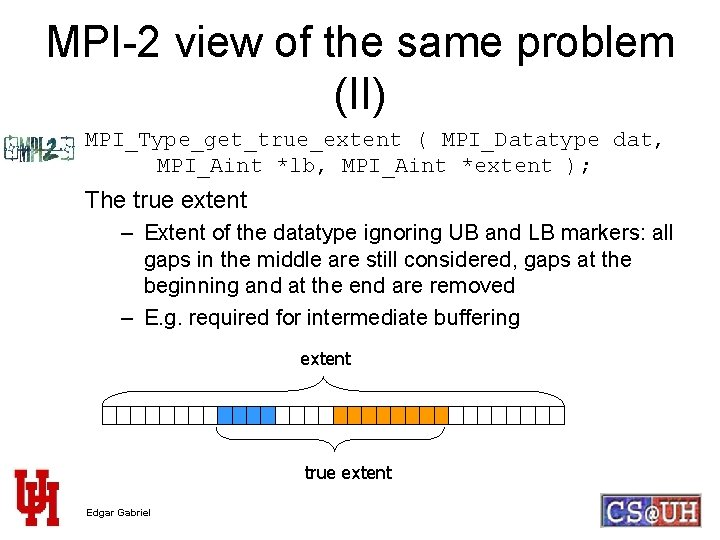MPI-2 view of the same problem (II) MPI_Type_get_true_extent ( MPI_Datatype dat, MPI_Aint *lb, MPI_Aint *extent ); The true extent – Extent of the datatype ignoring UB and LB markers: all gaps in the middle are still considered, gaps at the beginning and at the end are removed – E. g. required for intermediate buffering extent true extent Edgar GabrielDecoding MPI datatypes • An important question for library developers: – Given a datatype handle, can I determine how it was created – Given a datatype handle, can I determine what memory layout it describes ? • MPI-1: no • MPI-2: yes Edgar GabrielMPI_Type_get_envelope ( MPI_Datatype datatype, int *num_integers, int *num_addresses, int *num_datatypes, int *combiner ); • The combiner field returns how the datatype was created, e. g. – – – MPI_COMBINER_NAMED: MPI_COMBINER_CONTIGUOS: MPI_COMBINER_VECTOR: MPI_COMBINER_INDEXED: MPI_COMBINER_STRUCT: basic datatype MPI_Type_contiguous MPI_Type_vector MPI_Type_indexed MPI_Type_struct • The other fields indicate how large the integer-array, the datatype-array, and the address-array has to be for the following call to MPI_Type_get_contents Edgar GabrielMPI_Type_get_contents ( MPI_Datatype datatype, int max_integer, int max_addresses, int max_datatypes, int *integers, int *addresses, MPI_Datatype *dts); • Call is erroneous for a predefined datatypes • If returned data types are derived datatypes, then objects are duplicates of the original derived datatypes. User has to free them using MPI_Type_free • The values in the integer, addresses and datatype arrays are depending on the original datatype constructor • Type decoding functions available for MPICH 1. 2. 5 or MPICH 2 or LAM 7. 0. x Edgar GabrielExamples using MPI_Type_get_contents • e. g. for MPI_Type_struct count blocklengths[] displacements[] datatypes[] integers integers – integers] addresses–addresses[integers-1] dts – dts[integers-1] • e. g. for MPI_Type_contiguous count datatype integers dts • For the complete list, see the MPI-2 specification Edgar Gabriel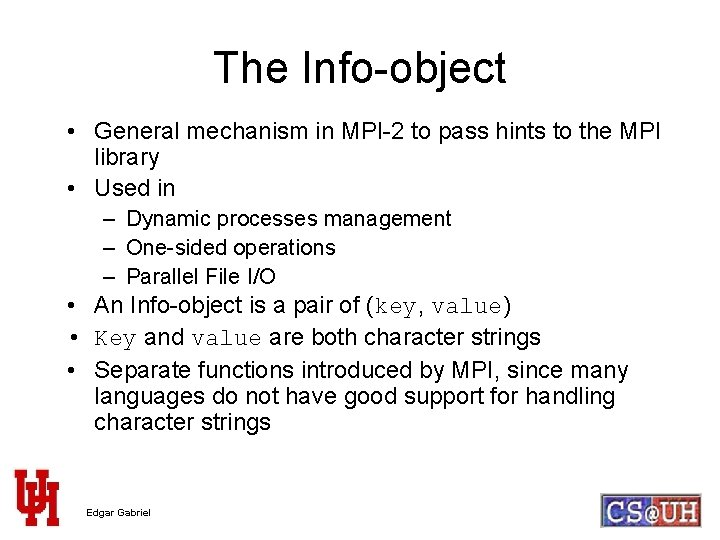The Info-object • General mechanism in MPI-2 to pass hints to the MPI library • Used in – Dynamic processes management – One-sided operations – Parallel File I/O • An Info-object is a pair of (key, value) • Key and value are both character strings • Separate functions introduced by MPI, since many languages do not have good support for handling character strings Edgar Gabriel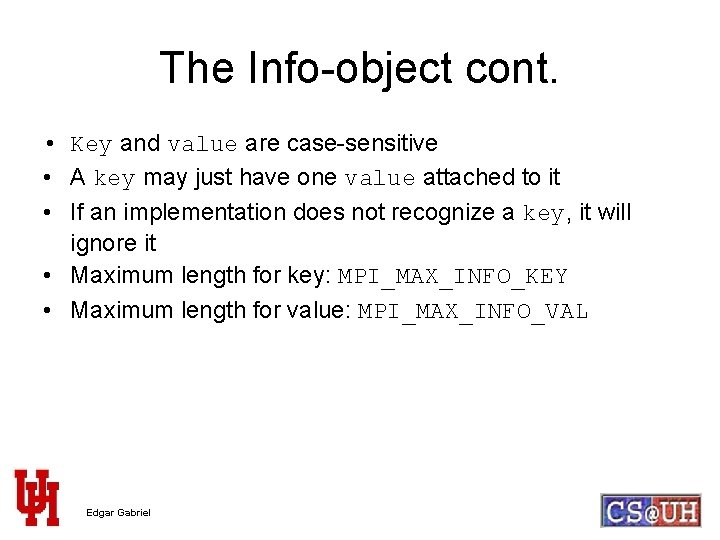The Info-object cont. • Key and value are case-sensitive • A key may just have one value attached to it • If an implementation does not recognize a key, it will ignore it • Maximum length for key: MPI_MAX_INFO_KEY • Maximum length for value: MPI_MAX_INFO_VAL Edgar Gabriel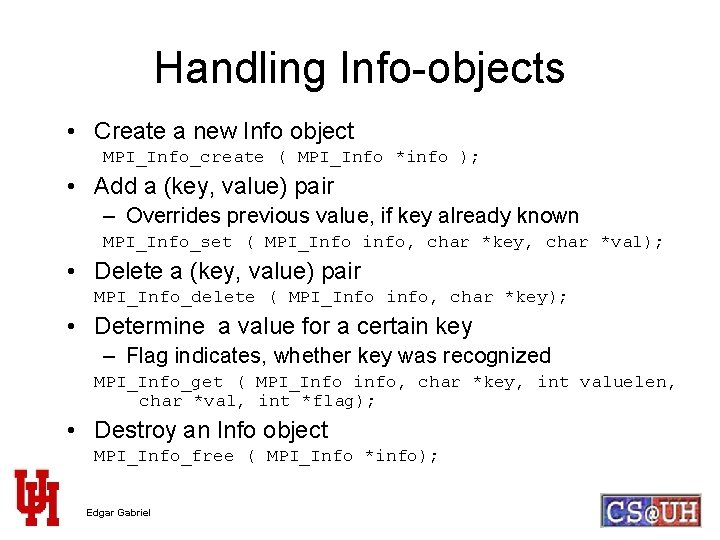Handling Info-objects • Create a new Info object MPI_Info_create ( MPI_Info *info ); • Add a (key, value) pair – Overrides previous value, if key already known MPI_Info_set ( MPI_Info info, char *key, char *val); • Delete a (key, value) pair MPI_Info_delete ( MPI_Info info, char *key); • Determine a value for a certain key – Flag indicates, whether key was recognized MPI_Info_get ( MPI_Info info, char *key, int valuelen, char *val, int *flag); • Destroy an Info object MPI_Info_free ( MPI_Info *info); Edgar Gabriel